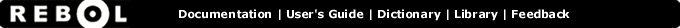# Lesser? - Function Summary

## Summary:

Returns TRUE if the first value is less than the second value.

## Usage:

lesser? value1 value2

## Arguments:

value1 - The value1 argument.

value2 - The value2 argument.

## Description:

Returns FALSE for all other values. The values must be of the same datatype, or an error will occur. For string-based datatypes, the sorting order is used for comparison with character casing ignored (uppercase = lowercase).

 ``` print lesser? "abc" "abcd" true```

 ``` print lesser? 12-june-1999 10-june-1999 false```

 ``` print lesser? 1.2.3.4 4.3.2.1 true```

 ``` print lesser? 1:30 2:00 true```

## Related:

<= - Returns TRUE if the first value is less than or equal to the second value.
<> - Returns TRUE if the values are not equal.
= - Returns TRUE if the values are equal.
> - Returns TRUE if the first value is greater than the second value.
>= - Returns TRUE if the first value is greater than or equal to the second value.
max - Returns the greater of the two values.
min - Returns the lesser of the two values.